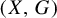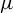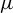Hostname: page-component-7d684dbfc8-8ckrc Total loading time: 0 Render date: 2023-09-30T01:19:02.068Z Has data issue: false Feature Flags: { "corePageComponentGetUserInfoFromSharedSession": true, "coreDisableEcommerce": false, "coreDisableSocialShare": false, "coreDisableEcommerceForArticlePurchase": false, "coreDisableEcommerceForBookPurchase": false, "coreDisableEcommerceForElementPurchase": false, "coreUseNewShare": true, "useRatesEcommerce": true } hasContentIssue false

# Packing topological entropy for amenable group actions

Published online by Cambridge University Press:  20 October 2021

## Abstract

Packing topological entropy is a dynamical analogy of the packing dimension, which can be viewed as a counterpart of Bowen topological entropy. In the present paper we give a systematic study of the packing topological entropy for a continuous G-action dynamical system$(X,G)$ , where X is a compact metric space and G is a countable infinite discrete amenable group. We first prove a variational principle for amenable packing topological entropy: for any Borel subset Z of X, the packing topological entropy of Z equals the supremum of upper local entropy over all Borel probability measures for which the subset Z has full measure. Then we obtain an entropy inequality concerning amenable packing entropy. Finally, we show that the packing topological entropy of the set of generic points for any invariant Borel probability measure$\mu$ coincides with the metric entropy if either$\mu$ is ergodic or the system satisfies a kind of specification property.

## MSC classification

Type
Original Article
Information
Ergodic Theory and Dynamical Systems , February 2023 , pp. 480 - 514

## Access options

Get access to the full version of this content by using one of the access options below. (Log in options will check for institutional or personal access. Content may require purchase if you do not have access.)

## References

Aaronson, J.. Relative complexity of random walks in random sceneries. Ann. Probab. 40(6) (2012), 24602482.CrossRefGoogle Scholar
Bowen, R.. Entropy for group endomorphisms and homogeneous spaces. Trans. Amer. Math. Soc. 153 (1971), 401414.CrossRefGoogle Scholar
Bowen, R.. Topological entropy for noncompact sets. Trans. Amer. Math. Soc. 184 (1973), 125136.CrossRefGoogle Scholar
Brin, M. and Katok, A.. On local entropy. Geometric Dynamics (Lecture Notes in Mathematics, 1007). Ed. Palis, J. Jr. Springer, Berlin, 1983, pp. 3038.CrossRefGoogle Scholar
Ceccherini-Silberstein, T. and Coornaert, M.. Cellular Automata and Groups. Springer-Verlag, Berlin, 2010.CrossRefGoogle Scholar
Chung, N. P. and Li, H.. Homoclinic groups, IE groups, and expansive algebraic actions. Invent. Math. 199(3) (2015), 805858.CrossRefGoogle Scholar
den Hollander, F. and Steif, J. E.. Random walk in random scenery: a survey of some recent results. Dynamics & Stochastics (Institute of Mathematical Statistics Lecture Notes—Monograph Series, 48), Institute of Mathematical Statistics, Beachwood, OH, 2006, pp. 5365.CrossRefGoogle Scholar
Dou, D. and Zhang, R.. A note on dimensional entropy for amenable group actions. Topol. Methods Nonlinear Anal. 51(2) (2018), 599608.Google Scholar
Feng, D. J. and Huang, W.. Variational principles for topological entropies of subsets. J. Funct. Anal. 263(8) (2012), 22282254.CrossRefGoogle Scholar
Hofmann, K.-H. and Stoyanov, L. N.. Topological entropy of group and semigroup actions. Adv. Math. 115 (1995), 5498.CrossRefGoogle Scholar
Jech, T.. Set Theory (Springer Monographs in Mathematics). Springer, Berlin, 2003.Google Scholar
Joyce, H. and Preiss, D.. On the existence of subsets of finite positive packing measure. Mathematika 42 (1995), 1524.CrossRefGoogle Scholar
Kalikow, S. A..$T,{T}^{-1}$ transformation is not loosely Bernoulli. Ann. of Math. (2) 115 (1982), 393409.CrossRefGoogle Scholar
Kerr, D. and Li, H.. Ergodic Theory: Independence and Dichotomies (Springer Monographs in Mathematics). Springer, Cham, 2016.CrossRefGoogle Scholar
Lindenstrauss, E.. Pointwise theorems for amenable groups. Invent. Math. 146 (2001), 259295.CrossRefGoogle Scholar
Mattila, P.. Geometry of Sets and Measures in Euclidean Spaces. Cambridge University Press, Cambridge, 1995.CrossRefGoogle Scholar
Ollagnier, J. M. and Pinchon, D.. The variational principle. Studia Math. 72(2) (1982), 151159.CrossRefGoogle Scholar
Ornstein, D. S. and Weiss, B.. Entropy and isomorphism theorems for actions of amenable groups. J. Anal. Math. 48 (1987), 1141.CrossRefGoogle Scholar
Pesin, Y. B.. Dimension Theory in Dynamical Systems (Contemporary Views and Applications) (Chicago Lectures in Mathematics). University of Chicago Press, Chicago, IL, 1997.CrossRefGoogle Scholar
Pfister, C.-E. and Sullivan, W. G.. On the topological entropy of saturated sets. Ergod. Th. & Dynam. Sys. 27(3) (2007), 929956.CrossRefGoogle Scholar
Stepin, A. M. and Tagi-Zade, A. T.. Variational characterization of topological pressure of the amenable groups of transformations. Dokl. Akad. Nauk 254(3) (1980), 545549.Google Scholar
Tricot, C.. Two definitions of fractional dimension. Math. Proc. Cambridge Philos. Soc. 91(1) (1982), 5774.CrossRefGoogle Scholar
Zhang, R.. Topological pressure of generic points for amenable group actions. J. Dynam. Differential Equations 30(4) (2018), 15831606.Google Scholar
Zhao, C., Chen, E., Hong, X. and Zhou, X.. A formula of packing pressure of a factor map. Entropy 19(10) (2017), Paper no. 526, 9 pp.CrossRefGoogle Scholar
Zheng, D. and Chen, E.. Bowen entropy for actions of amenable groups. Israel J. Math. 212 (2016), 895911.CrossRefGoogle Scholar
Zheng, D. and Chen, E.. Topological entropy of sets of generic points for actions of amenable groups. Sci. China Math. 61(5) (2018), 869880.CrossRefGoogle Scholar
Zhou, X., Chen, E. and Cheng, W.. Packing entropy and divergence points. Dyn. Syst. 27(3) (2012), 387402.CrossRefGoogle Scholar